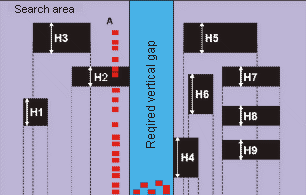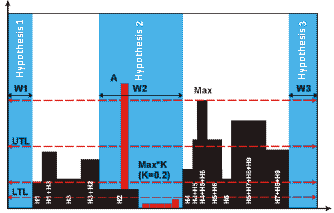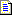English (English)

# Hypotheses for White Gap elements

The program formulates White Gap hypotheses by creating the histograms of candidate objects.

By default, the program looks for a White Gap between Any Text objects. To look for a White Gap between other types of object (e.g. between Separators), you need to write a corresponding constraint on the Properties dialog box of the White Gap element (Advanced tab, Advanced pre-search relations field). For example, if you need to find a White Gap in an area where all types of objects may occur, you need to write the following expression: Type: PictureObject + SeparatorObject+ AnyText + PunctuationMark + CheckMarkObject;.

## A histogram is created as follows:

The program projects all the objects of a certain type which have been detected in the search area on the horizontal or vertical axis. The projection is a sum total of the objects' widths or heights. When looking for a horizontal gap, the program creates a projection on the vertical axis. When looking for a vertical gap, the program creates a projection on the horizontal axis; when looking for a horizontal gap, the program creates a projection on the vertical axis. The linear size of each object of the given size is added to the projection. For example, to find a vertical White Gap among the text objects, the program sums up the heights of all the text objects located in the search area above a particular point on the horizontal axis and intersecting the search area of the element.

Then the program looks for regions where the height of the histogram is less than a particular value. These regions will correspond to areas in which the number of objects is relatively small and their projection is less than a certain pre-defined value. The program must allow a certain number of objects to be present in the White Gap because real images often contain speckles and other noise introduced during scanning and which must be ignored when looking for gaps between columns or paragraphs. Background noise does not much affect the overall profile.

Suppose we have text objects H1, H2,..., H9 in the search area. In the figure below, these objects are highlighted in black. Let the search contain other types of objects (highlighted in red).To find the vertical White Gap, we need to find the sum up the projections of the text objects on the horizontal axis. The resulting histogram is shown in the figure below. You can see that non-text objects are ignored in the histogram.Next, we need to find the histogram Maximum (marked as Max in the figure). The value of the maximum level is then multiplied by the value set in Threshold coefficient (%) (K=0.2). The result is the maximum allowed level of the White Gap (marked as White Gap threshold in the figure). If the resulting White Gap threshold >0, other objects may be present in the area of the White Gap.

Once the White Gap threshold has been calculated, it is compared with the values set in Lower threshold limit and Upper threshold limit. If White Gap threshold < Lower threshold limit, the White Gap threshold is assigned the value of the Lower threshold limit and this value will be used to look for the White Gap. If White Gap threshold > Upper threshold limit, the White Gap threshold is assigned the value of the Upper threshold limit.

Next, the heights on the histogram are compared with the White Gap threshold in order to find areas where the level of the histogram is less than the White Gap threshold.

The Min width/height property sets the minimum absolute width of the White Gap. If the value is W2, the two other hypotheses will be discarded.

A White Gap hypothesis has the following properties:

 Property Description Element name The full name of the element. Page The number of the page on which the element was detected. Surrounding rect The coordinates of the rectangle which surrounds the region of the hypothesis. Width The width of the region of the hypothesis. Height The height of the region of the hypothesis. Orientation The orientation of the detected White Gap. Histogram maximum in search area The peak of the histogram in the search area. White Gap threshold The point in the histogram below which the program starts formulating White Gap hypotheses. Histogram maximum within hypothesis The peak of the histogram maximum within the hypothesis. Detected Shows whether the object described by the element has been found (true) or whether a null hypothesis has been formulated (false). From the best path Shows whether the found hypothesis belongs to the best path in the tree of hypotheses (true) or not (false). Pre-search quality How well the hypothesis matches the properties of the element specified by the settings in the Properties dialog box and by the code in the Advanced pre-search relations. Post-search quality The quality of the hypothesis after the conditions in the Advanced post-search relations field have been applied. Chain quality The quality of the chain of hypotheses, from the first subelement of the group to the current subelement. Chain quality is calculated by multiplying the qualities of all the subelements in the chain and is used to compare rival chains of hypotheses.

#### More:White GapSearch areaAdditional search constraints

25.05.2023 7:55:03